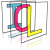Image Component Library (ICL)
icl::utils::Function< R, A, B, C > Struct Template Reference

The General Function Template. More...

`#include <Function.h>`

## Public Member Functions

Function ()
Empty constructor (implementation will become null) More...

Function (FunctionImpl< R, A, B, C > *impl)
Constructor with given Impl. More...

Function (icl::utils::SmartPtr< FunctionImpl< R, A, B, C > >impl)
Constructor with given SmartPtr<Impl> More...

Function (R(*global_function)(A, B, C))
Constructor from given global function (for implicit conversion) More...

operator() (A a, B b, C c) const
function operator (always const) More...

operator bool () const
checks wheter the implemnetation is not null More...

operator Function< R, A, B, C > () const

## Public Attributes

icl::utils::SmartPtr< FunctionImpl< R, A, B, C > > impl
Implementation. More...

## Detailed Description

### template<class R = void, class A = NO_ARG, class B = NO_ARG, class C = NO_ARG> struct icl::utils::Function< R, A, B, C >

The General Function Template.

The Function class can be used as a generic functor that can have one of these backends:

• A global function call
• A member function call
• A call to an objects function operator (i.e. it wrapps a functor)
• An arbitrary implementation by wrapping a custom implementation of FunctionImpl<R,A,B>

This class should not be used directly! Use the overloaded icl::utils::function - template instead. Functions can be copied as objects. Internally, a SmartPointer is used to manage the actual function implementation.

The Function class template is specialized for functions with less than two parameters. In this case the Function's function-operator() also has less parameters.

FUNCTION_SECTION

## ◆ Function() [1/4]

template<class R = void, class A = NO_ARG, class B = NO_ARG, class C = NO_ARG>
 icl::utils::Function< R, A, B, C >::Function ( )
inline

Empty constructor (implementation will become null)

## ◆ Function() [2/4]

template<class R = void, class A = NO_ARG, class B = NO_ARG, class C = NO_ARG>
 icl::utils::Function< R, A, B, C >::Function ( FunctionImpl< R, A, B, C > * impl )
inline

Constructor with given Impl.

## ◆ Function() [3/4]

template<class R = void, class A = NO_ARG, class B = NO_ARG, class C = NO_ARG>
 icl::utils::Function< R, A, B, C >::Function ( icl::utils::SmartPtr< FunctionImpl< R, A, B, C > > impl )
inline

Constructor with given SmartPtr<Impl>

## ◆ Function() [4/4]

template<class R = void, class A = NO_ARG, class B = NO_ARG, class C = NO_ARG>
 icl::utils::Function< R, A, B, C >::Function ( R(*)(A, B, C) global_function )
inline

Constructor from given global function (for implicit conversion)

This constructor can be used for implicit conversion. Where a Function<R,A,B> is expected, you can simply pass a global function of type R(*function)(A,B)

## ◆ operator bool()

template<class R = void, class A = NO_ARG, class B = NO_ARG, class C = NO_ARG>
 icl::utils::Function< R, A, B, C >::operator bool ( ) const
inline

checks wheter the implemnetation is not null

## ◆ operator Function< R, A, B, C >()

template<class R = void, class A = NO_ARG, class B = NO_ARG, class C = NO_ARG>
 icl::utils::Function< R, A, B, C >::operator Function< R, A, B, C > ( ) const
inline

## ◆ operator()()

template<class R = void, class A = NO_ARG, class B = NO_ARG, class C = NO_ARG>
 R icl::utils::Function< R, A, B, C >::operator() ( A a, B b, C c ) const
inline

function operator (always const)

This is const, since the creator template icl::utils::function will automatically create the correct implementation

## ◆ impl

template<class R = void, class A = NO_ARG, class B = NO_ARG, class C = NO_ARG>
 icl::utils::SmartPtr > icl::utils::Function< R, A, B, C >::impl

Implementation.

The documentation for this struct was generated from the following file:
• /Users/alneuman/vm/icl/ICLUtils/src/ICLUtils/Function.h